# Area and Perimeter of a Rectangle Worksheets

###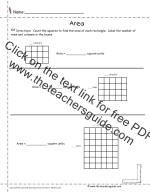Area Count the Square Units

Students count square units to find the area of a rectangle.

###Area Skip Count the Square Units

Students Skip Count square units to find the area of a rectangle.

###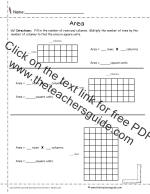Area Muliply the Square Units

Students multiply the rows and columns of square units to find the area of a rectangle.

###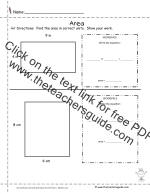Area Multiply the Side Lengths

Students multiply the side lengths to find the area of a rectangle.

###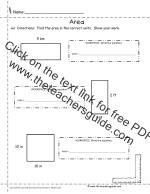Area Multiply the Side Lengths 2

Students count square units to find the area of a rectangle.

###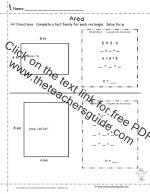Area Solve for Unknown Length or Width

Students solve for the unknown length or width.

###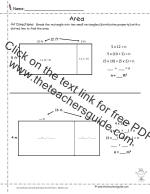Area of a Rectangle Distributive Property

Students use the distributive property area model.

###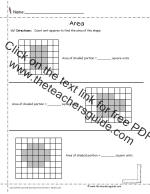Area Shaded Shapes

Students count square units to find the area of a shaded shape.

###Area Make Two Rectangles

Students make a shape into two rectangles to find the area.

###Area Count the Square Units

Students use grid cues to find the area of the shaded shape.

###Area of Shaded Shape by Subtracting With Blocks

Students find the area of a shade shape by making a full rectangle and subtracting.

###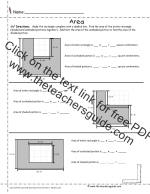Area of Shaded Shape by Subtracting Without Blocks

Students find the area of a shade shape by making a full rectangle and subtracting.

###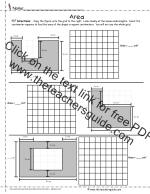Area of Shaded Shape by Subtracting Copy to Grid

Students find the area of a shade shape copying the shape to a grid and counting the unit squares.

###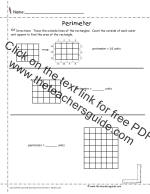Perimeter Counting Outer Edges

Students find perimeter of a rectangle by counting the outer edges of outer square units.

###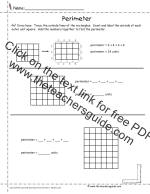Perimeter Adding Outer Edges

Students find perimeter of a rectangle by adding the outer edges of outer square units.

###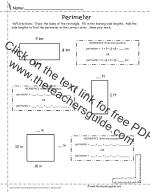Perimeter Adding Side Lengths

Students find the area of a shade shape by making a full rectangle and subtracting.

###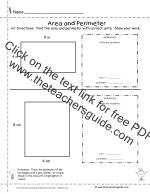Area and Perimeter Side Lengths

Students find the area and perimeter of a rectangle by working with side lengths.

###Area of and Perimeter Side Lengths 2

Students find the area and perimeter of a rectangle by working with side lengths.

###Area and Perimeter Word Problems

Students solve word problems involving area and perimeter.

###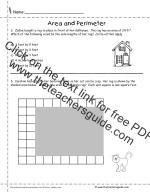Area and Perimeter Word Problems

Students solve word problems involving area and perimeter.

###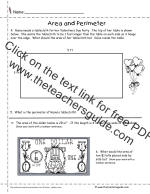Area and Perimeter Word Problems

Students solve word problems involving area and perimeter.

###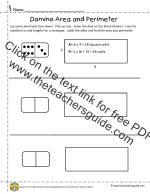Area and Perimeter Dominoes

Students use dominoes to calculate area.